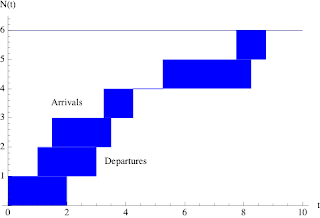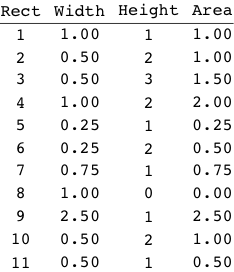## Sunday, April 28, 2013

### Visual Proof of Little's Law Reworked

Back in early March, when I was at the Hotsos Symposium on Oracle performance, I happened to end up sitting next to Alain C. at lunch. He always attends my presentations, especially on USL scalability analysis. During our lunchtime conversation, he took out his copy of Analyzing Computer System Performance with Perl::PDQ and opened it at the section on the visual proof for Little's law. Alain queried (query ... Oracle ... Get it?) whether the numbers really added up the way they are shown in the diagrams. It did look like there could be a discrepancy but it was too difficult to reanalyze the whole thing over lunch.

I later recalled that I had done the original calculations in Excel a long time ago for the 1998 edition of The Practical Performance Analyst and those diagrams were done in Powerpoint. Such were the authoring tools I had at that time. (blush) Subsequently, those calculations were not reviewed for the 2nd edition in 2011 and the visual mistake was not caught. Following up on the lunchtime discussion, I decided to start from scratch and reproduce the entire argument using the exacting capabilities of Mathematica, instead of Excel.

It turns out that Alain was right. His observation have now been added to the corrigenda page.

Section 4.5.2 Visual Proof shows the instantaneous number N(t) of arrivals and departures at a queueing facility as a function of time t. The difference between the arrival and departure counts (one of the 3 fundamental performance metrics) is shown as the dark blue region in Fig. 4.9Figure 4.9. Same as the original

The visual proof of Little's law relies on demonstrating that the total blue area calculated using the horizontally oriented rectangles in Fig. 4.10 is the same as that calculated using the vertically oriented rectangles in Fig. 4.11. Even using Mathematica, this is much trickier than it looks.Figure 4.10: Same as the original diagram but with vertical rectangle boundaries shown

The results for Fig. 4.10 are summarized in Table 4.2.Table 4.2. Same as the original but explicitly including the zero area of the 5th segment

The total height is exactly 6 units, consistent with Fig. 4.10. The total area is 11.00 units, which is also consistent with the book text. The 2 decimal digits of accuracy is merely for later comparison. Next, we calculate the vertical rectangles in Fig. 4.11.Figure 4.11. Corrected diagram

The results for Fig. 4.11 are summarized in Table 4.3.Table 4.3. Corrected values

The total area is also 11.00 units, but the contribution of some vertical rectangles is different from those in the original text. Consequently, the total width is 8.75 time units, not 8.00 time units as shown in the book Table 4.3.

Thanks, Alain. Well spotted!